# Hopf orders, applications of

(diff) ← Older revision | Latest revision (diff) | Newer revision → (diff)

Letbe a finite extension of the-adic rationals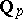endowed with the-adic valuationwithand letbe its ring of integers (cf. also Extension of a field; Norm on a field;-adic number). Letbe the ramification index ofinand assume thatcontains a primitiverd root of unity. If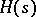is an-Hopf order in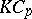(cf. Hopf order), then the group scheme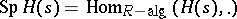can be resolved, i.e., involved in a short exact sequence of group schemes(taken in the flat topology). Here,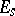and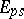are represented by the-Hopf algebrasand, respectively (an indeterminate). One uses this short exact sequence in the long exact sequence in cohomology to construct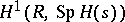, which is identified with the group of-Galois extensions of(cf. also Galois extension). One has, where the classcorresponds to the isomorphism class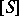of the-Galois extension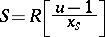with(see [a2]). Moreover, if,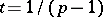, is the dual of the Larson order(cf. Hopf order), then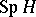can be involved in the short exact sequence of group schemes, where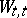andare represented by the-Hopf algebras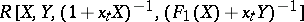and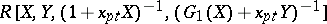for appropriate polynomials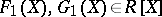, respectively (see [a3]). Work has been completed to obtain a resolution ofwhen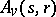is an arbitrary-Hopf order in(see [a4]). This yields (via the long exact sequence in cohomology) a characterization of all-Galois extensions. In general, ifis an-Hopf order in, thenis an-Galois extension if and only ifis an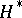-Galois algebra (see [a1]).

Examples of-Galois extensions whereis a Tate–Oort–Larson order incan be recovered using the corresponding classification theorem. For example, if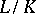is a Kummer extension of prime degree, then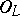is an-Galois algebra if and only if the ramification numberofsatisfies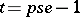(see [a1]). Therefore,is an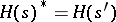-Galois extension, thus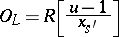for some parameter. Knowledge of Tate–Oort Galois extensions can also be used to characterize the ring of integers of certain degree-extensions. For example, if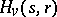,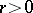, is a Greither order with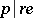and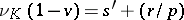, then there exists a finite extensionso thatis an-Galois algebra. In this case,is of the form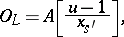whereis an element inandis an-Galois extension (see [a2]).

How to Cite This Entry:
Hopf orders, applications of. Encyclopedia of Mathematics. URL: http://encyclopediaofmath.org/index.php?title=Hopf_orders,_applications_of&oldid=42724
This article was adapted from an original article by R.G. Underwood (originator), which appeared in Encyclopedia of Mathematics - ISBN 1402006098. See original article